2-room apartment, 40.5 m2, 2/2 floor.2-room apartment, 43 m2 1/1 FL.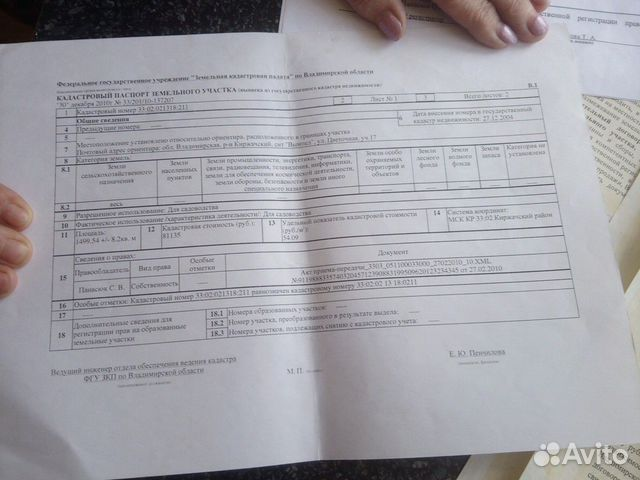Plot 15 hundred. (IH)Plot of 18 hundred. (IH)The house is 57.3 m2, plot 14 SOT.1 KOMN. kV.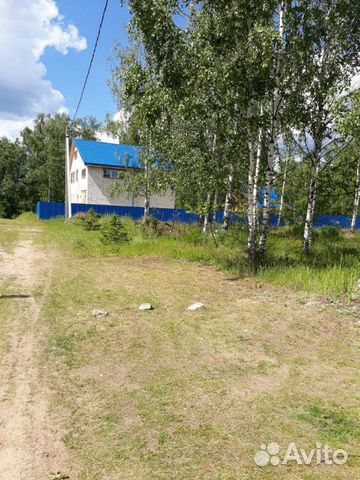Plot 16 hundred. (IH)The plot is 11 hundred. (IH)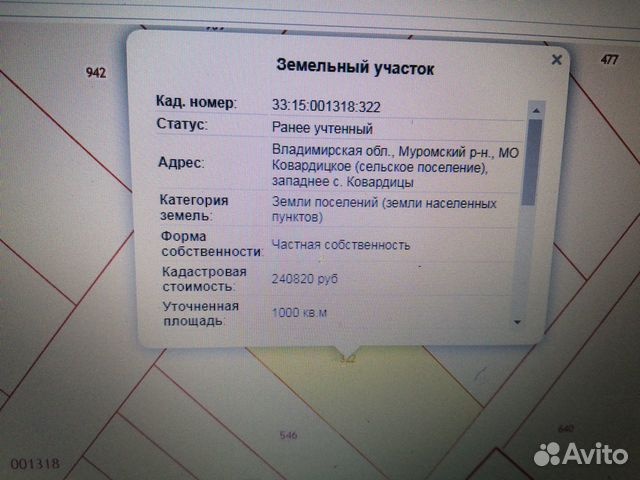A plot of 10 hundred. (IH)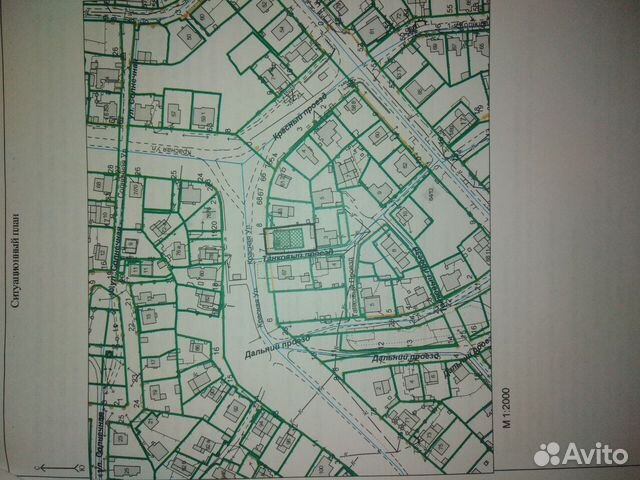A plot of 9 cells. (IH)A plot of 10 hundred. (IH)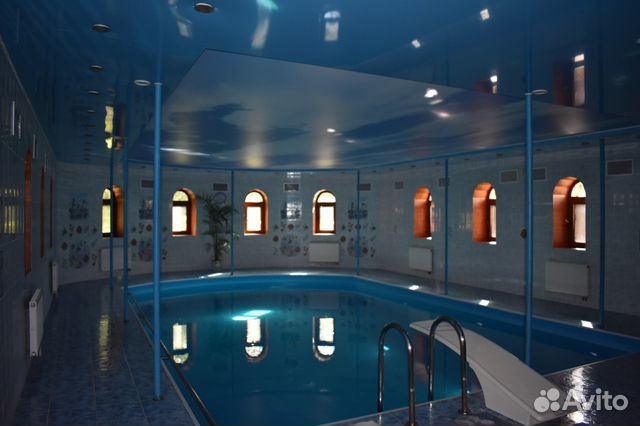1000 m2 cottage on a plot of 40 SOT.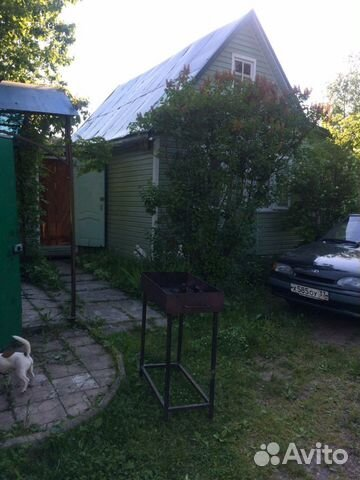Cottage 36 m2 on a plot of 7 hundred.